# Bihar Board Class 10th Maths Solutions Chapter 6 Triangles Ex 6.6

Bihar Board Class 10th Maths Solutions Chapter 6 Triangles Ex 6.6 Textbook Questions and Answers.

## BSEB Bihar Board Class 10th Maths Solutions Chapter 6 Triangles Ex 6.6Question 1.
In the figure, PS is the bisector of ∠QPR of ∆ PQR. Prove that $$\frac { QS }{ SR }$$ = $$\frac { PQ }{ PR }$$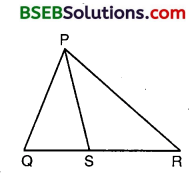Solution:
Given : PQR is a triangle and PS is the bisector of ∠QPR meeting QR at S.
∴ ∠QPS = ∠SPR
To Prove : $$\frac { QS }{ SR }$$ = $$\frac { PQ }{ PR }$$
Construction : Draw RT || SP to cut QP produced at T.
Proof :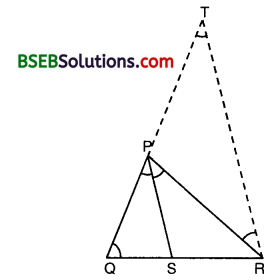Since PS || TR and PR cuts them, hence we have :
∠SPR = ∠PRT … (1) [Alternate ∠S]
and ∠QPS = ∠PTR … (2) [Corresponding ∠S]
But, ∠QPS = ∠SPR [Given]
∴ ∠PRT = ∠PTR [From (1) and (2)]
So, PT = PR … (3) [∵ Sides opp. to equal ∠S are equal]
Now, in ∆ QRT, we have
SP || RT [By Construction]
∴ $$\frac { QS }{ SR }$$ = $$\frac { PQ }{ PT }$$ [By Basic Proportionality Theorem]
or $$\frac { QS }{ SR }$$ = $$\frac { PQ }{ PR }$$ [From (3)]

Question 2.
In the figure, D is a point on hypotenuse AC of ∆ ABC, BD ⊥ AC, DM ⊥ BC and DN ⊥ AB. Prove that(i) DM² = DN.MC
(ii) DN² = DM.AN
Solution:
We have : AB ⊥ BC and DM ⊥ BC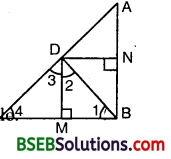So, AB || DM
Similarly, we have :
BC ⊥ AB and DN ⊥ AB.
So, CB || DN
Hence, quadrilateral BMDN is a rectangle.
BM = ND

(ii) In ∆ BMD, we have :
∠1 + ∠BMD + ∠2 = 180°
or ∠1 + 90° + ∠2 = 180° or ∠1 + ∠2 = 90°
Similarly, in ∆ DMC, we have :
∠3 +∠4 = 90°
Since BD ⊥ AC, therefore
∠2 + ∠3 = 90°
Now, ∠1 + ∠2 = 90° and ∠2 + ∠3 = 90°
∴ ∠1 + ∠2 = ∠2 + ∠3
So, ∠1 = ∠3
Also, ∠3 +∠4 = 90° and ∠2 + ∠3 = 90°
∴ ∠3 +∠4 = ∠2 + ∠3 So, ∠2 =∠4
Thus, in As BMD and DMC, we have :
∠1 = ∠3 and ∠2 =∠4
∴ By AA criterion of similarity, we have :
∆ BMD ~ ∆ DMC
So, $$\frac { BM }{ DM }$$ = $$\frac { MD }{ MC }$$
or $$\frac { DN }{ DM }$$ = $$\frac { DM }{ MC }$$ [∵BM = ND]
so, DM² = DN.MC

(ii) Proceeding as in (i), we can prove that
∆ BMD ~ ∆ DMC
So, $$\frac { BN }{ DN }$$ = $$\frac { ND }{ NA }$$
or $$\frac { DM }{ DN }$$ = $$\frac { DN }{ AN }$$ [∵BN = DM]
so, DN² = DM.ANQuestion 3.
In the figure, ABC is a triangle in which ∠ABC >90° and AD ⊥ CB produced. Prove that AC² = AB² + BC² + 2BC.BD.Solution:
Given : ABC is a triangle in which ∠ABC > 90° and AD ⊥ CB produced.
To Prove : AC² = AB² + BC² + 2BC.BD.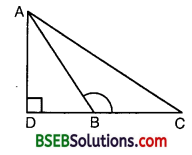Proof : Since ∆ ADB is a right triangle, right angled at D, therefore, by Pythagoras theorem, we have :
AB² = AD² + DB² … (1)
Again, ∆ ADC is a right triangle, right angled at D.
Therefore, by Pythagoras theorem, we have
or AC² =AD² + (DB + BC)2
or AC² =AD² + DB²+ BC² + 2DB.BC
or AC² = (AD² + DB²) + BC² + 2BC.BD
or AC² = AB² +BC² + 2BC.BD [Using (1)]
which proves the required result.

Question 4.
In the figure, ABC is a triangle in which ∠ABC < 90° and AD ⊥ BC. Prove that
AC² = AB² + BC² – 2BC.BD.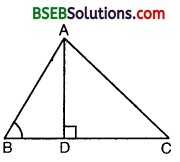Solution: Given : ABC is a triangle in which ∠ABC < 90° and AD ⊥ BC.
To Prove : AC² = AB² + BC² – 2BC . BD.
Proof : Since ∆ ADB is a right triangle, right angled at D, therefore, by Pythagoras theorem, we have :
AB² = AD² + BD² … (1)
Again, ∆ ADC is a right triangle, right angled at D. Therefore, by Pythagoras theorem, we have :
or AC² = AD² + (BC – BD)²
or AC² = AD² + (BC² + BD² – 2BC.BD)
or AC² = (AD² + BD²) + BC² – 2BC.BD
or AC² = AB² + BC² – 2BC.BD [Using (1)]
which proves the required result.

Question 5.
In the figure, AD is a median of a triangle ABC and AM ⊥ BC. Prove that(i) AC² = AD² + BC . DM + ($$\frac { BC }{ 2 }$$)²
(ii) AB² = AD² – BC . DM + ($$\frac { BC }{ 2 }$$)²
(iii) AC² + AB² = 2AD² + $$\frac { 1 }{ 2 }$$BC².
Solution:
Since ∠AMD = 90°, therefore ∠ADM < 90° and ∠ADC > 90°.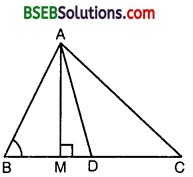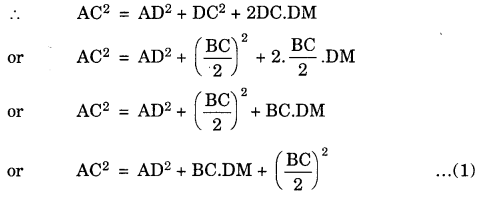(ii) In ∆ ABD, ∠ADB is an acute angle.(iii) From (1) and (2), we get
AB² + AC² = 2AD² + $$\frac { 1 }{ 2 }$$BC².Question 6.
Prove that the sum of the squares of the diagonals of a parallelogram is equal to the sum of the squares of its sides.
Solution:
We know that if AD is a median of ∆ ABC, then AB² + AC² = 2AD² + $$\frac { 1 }{ 2 }$$BC²
Since the diagonals of a parallelogram bisect each other, therefore BO and DO are medians of ∆s ABC and ADC respectively.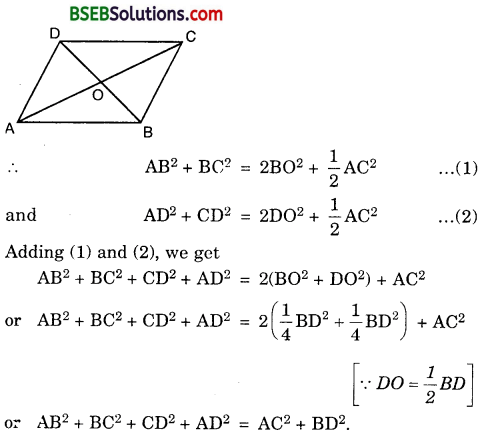Question 7.
In the figure, two chords AB and CD intersect each other at the point P. Prove that(i) ∆ APC ~ ∆ DPB
(ii) AP.PB = CP.DP
Solution:
(i) In ∆s APC and DPB, we have
∠APC = ∠DPB
∠CAP = ∠BDP [Angles in the same segment of a circle are equal]
∴ By AA criterion of similarity, we have :
∆ APC ~ ∆ DPB.

(ii) Since ∆ APC ~ ∆ DPB, therefore
∴ $$\frac { AP }{ DP }$$ = $$\frac { CP }{ PB }$$ or AP.PB = CP.DP

Question 8.
In the figure, two chords AB and CD of a circle intersect each other at the point P (when produced) outside the circle. Prove that(i) ∆ PAC ~ ∆ PDB
(ii) PA.PB = PC.PD
Solution:
(i) In ∆s PAC and PDB, we have :
∠APC = ∠BPD [Common]
∠PAC = ∠PDB [∵∠BAC = 180° – ∠PAC and ∠PDB = ∠CDB = 180° – ∠BAC = 180° – (180° – ∠PAC) = ∠PAC]
∴ By AA criterion of similarity, we have
∆ PAC ~ ∆ PDB

(ii) Since ∆ PAC ~ ∆ PDB, therefore
$$\frac { PA }{ PD }$$ = $$\frac { PC }{ PB }$$ OR PA.PB = PC.PD

Question 9.
In the figure, D is a point on side BC of ∆ ABC such that $$\frac { BD }{ CD }$$ = $$\frac { AB }{ AC }$$. Prove that AD is the bisector of ∠BAC.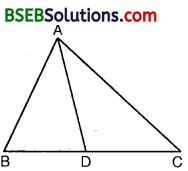Solution:
Given : ABC is a triangle and D is a point on BC such that
$$\frac { BD }{ CD }$$ = $$\frac { AB }{ AC }$$
To Prove : AD is the bisector of ∠BAC.
Construction : Produce BA to E such that AE = AC. Join CE.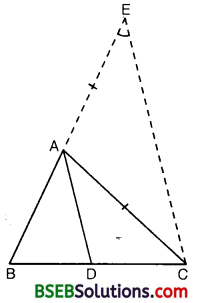Proof : In ∆ AEC, since AE = AC, hence
∠AEC = ∠ACE …(1) [∵ Angles opp. to equal sides of a A are equal]
Now, $$\frac { BD }{ CD }$$ = $$\frac { AB }{ AC }$$ [Given]
So, $$\frac { BD }{ CD }$$ = $$\frac { AB }{ AE }$$ [∵ AE = AC, construction]
∴ By converse of Basic Proportionality Theorem, we have :
DA || CE
Now, since CA is a transversal, we have :
∠BAD = ∠AEC … (2) [Corresponding ∠S]
and ∠DAC = ∠ACE … (3) [.Alternate angles]
Also, ∠AEC – ∠ACE [From (1)]
Hence, ∠BAD= ∠DAC [From (2) and (3)]Question 10.
Nazima is fly fishing in a stream. The tip of her fishing rod is 1.8 m above the surface of the water and the fly at the end of the string rests on the water 3.6 m away and 2.4 m from a point directly under the tip of the rod. Assuming that her string (from the tip of her rod to the fly) is taut, how much string does she have out (see figure) ? If she pulls in the string at the rate of 5 cm per second, what will be the horizontal distance of the fly from her after 12 seconds ?Solution:
In fact, we want to find. AC.By Pythagoras Theorem, we have :
AC² – (2.4)² + (1.8)²
or AC² = 5.76 + 3.24
= 9.00
or AC = 3 m
∴ Length of string she have out = 3m.
Length of the string pulled at the rate of 5 cm/sec in 12 seconds
= (5 x 12) cm
= 60 cm = 0.60 m
Remaining string left out
= (3 – 0.6) m
= 2.4 m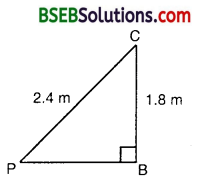In 2nd case, let us find PB.
PB² = PC² – BC²
= (2.4)² – (1.8)²
= 5.76 – 3.24 = 2.52
or PB = $$\sqrt{2.52}$$ = 1.59 (nearly)
Hence, the horizontal distance of the fly from Nazima after 12 seconds
= (1.59 + 1.2) m
= 2.79 m (nearly).